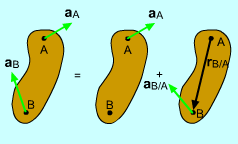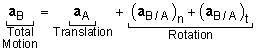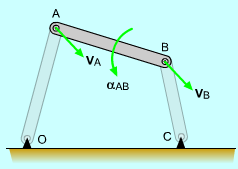Ch 5. Rigid Body General Motion Multimedia Engineering Dynamics Fixed Axis Rotation Plane Motion Velocities Zero Velocity Point Plane Motion Accelerations Multiple Gears Rot. Coord. Velocities Rot. Coord. Acceleration
 Chapter - Particle - 1. General Motion 2. Force & Accel. 3. Energy 4. Momentum - Rigid Body - 5. General Motion 6. Force & Accel. 7. Energy 8. Momentum 9. 3-D Motion 10. Vibrations Appendix Basic Math Units Basic Equations Sections Search eBooks Dynamics Fluids Math Mechanics Statics Thermodynamics Author(s): Kurt Gramoll ©Kurt GramollDYNAMICS - THEORYRelative Acceleration Equation

Relative acceleration equation can be derived from the the relative velocity equation,

vB = vA + vB/A

Differentiate with respect to time to give

ĀĀĀĀĀĀĀĀĀĀĀĀĀĀĀ     dvB/dt =dvA/dt + dvB/A/dt

 aB = aA + aB/A

Just like the relative velocity equation, the relative acceleration equation can be separated into linear motion and angular motion. However, it is important to note that the angular motion has two components, tangential and normal acceleration.3-Bar Acceleration Motion

Each rotation term can be written as cross products, giving

aB = aA + ωAB × (ωAB × rAB) + αAB × rAB

This form shows that the relative acceleration is composed of the translating motion of base point A and the rotating motion of point B about A.

For plane motion, the normal rotation terms can be simplified as -ω2r giving

aB = aA - ω2rAB + α × rAB

Another way to write the relative acceleration equation is

aB = aA - ω2ren + αret

Practice Homework and Test problems now available in the 'Eng Dynamics' mobile app
Includes over 400 problems with complete detailed solutions.
Available now at the Google Play Store and Apple App Store.